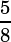Hello Mike!
If a and b are integers such that a+b<1000 and a/b=0.625, what is the greatest possible value of b?
Do we have to convert a/b to a fraction to find the answer? Is there any other way?
Thank you

I think most calculators will convert to a fraction for you, and yes, that is the best way to do this. .625 is, so ab will be some multiple of 5 + 8 = 13. What’s the biggest multiple of 13 that’s still under 1000?

1000/13 = 76.923… so we know that 76(13) = 988 is the biggest multiple of 13 under 1000.

The greatest value of b, then, is 76(8) = 608.